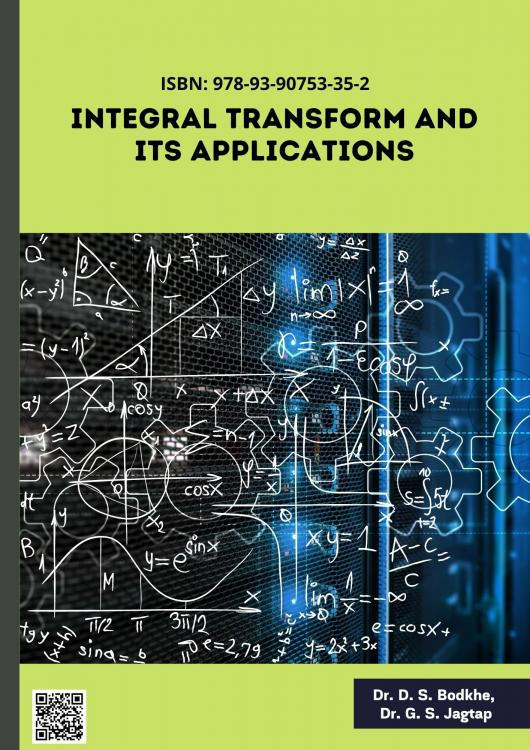# INTEGRAL TRANSFORM AND ITS APPLICATIONS

## Authors

Dr. D. S. Bodkhe
Head, Department of Mathematics, Anandrao Dhonde Alias Babaji Mahavidyalaya , Kada, India
Dr. G. S. Jagtap
Associate Professor, Department of mathematics, Anandrao Dhonde Alias Babaji Mahavidyalaya, Kada, India

## Keywords:

INTEGRAL TRANSFORM, FOURIER TRANSFORMS, LAPLACE TRANSFORMS, APPLICATIONS OF LAPLACE TRANSFORMS, SUMUDU TRANSFORMS, APPLICATIONS OF SUMUDU TRANSFORMS

## Synopsis

We present a novel complicated integral transform, the complex SEE transform, in this book. The features of this transform are studied. This complex integral transform is also used to simplify the core issue to a simple algebraic equation. The solution to this basic issue may then be determined by solving this algebraic equation and applying the inverse of this complex integral transform. Finally, the complex integral transform is employed to solve higher order ordinary differential equations. Also, we present several key engineering and physics applications. This chapter of the course teaches two incredibly effective ways to solve differential equations: the Fourier and the Laplace transforms. Beside its practical application, the Fourier transform is also of vital significance in quantum physics, establishing the relationship between the position and momentum representations of the Heisenberg commutation relations. An integral transform is valuable if it helps one to convert a difficult issue into a simpler one. The transforms we will be learning in this portion of the course are largely used to solve differential and, to a lesser degree, integral equations. The theory of Fourier series and integrals has always had considerable challenges and requires a huge mathematical apparatus in dealing with concerns of convergence. It encouraged the creation of techniques of summation, albeit they did not lead to a wholly adequate solution of the issue. ... For the Fourier transform, the inclusion of distributions (thus, the space S) is inevitable either in an explicit or hidden form. ... As a consequence one may acquire everything that is wanted from the point of view of the continuity and inversion of the Fourier transform.